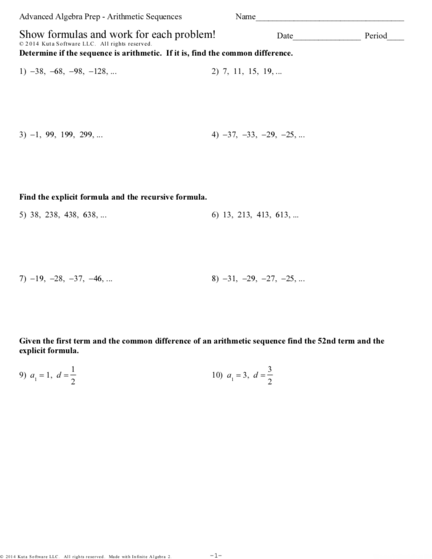# Arithmetic Sequences worksheetContributed by:Arithmetic Sequences worksheet. In an Arithmetic Sequence, the difference between one term and the next is a constant.
1. Advanced Algebra Prep - Arithmetic Sequences Name___________________________________
Show formulas and work for each problem! Date________________ Period____
©f h2N0Q1T4C AKCuztPat eSJoHfotswNaYrbeA 3L2LdCw.V t 2AalQl7 1rNiugchItrs5 or9eCske7r4v8eodZ.i
Determine if the sequence is arithmetic. If it is, find the common difference.
1) −38, −68, −98, −128, ... 2) 7, 11, 15, 19, ...
3) −1, 99, 199, 299, ... 4) −37, −33, −29, −25, ...
Find the explicit formula and the recursive formula.
5) 38, 238, 438, 638, ... 6) 13, 213, 413, 613, ...
7) −19, −28, −37, −46, ... 8) −31, −29, −27, −25, ...
Given the first term and the common difference of an arithmetic sequence find the 52nd term and the
explicit formula.
1 3
9) a1 = 1, d = 10) a1 = 3, d =
2 2
©M w2t011V4E 4KnuEt7a9 GSZogflt6wZarrReI 4LtLNCH.A 3 HA5lXlY FrYiggPhct3su 0rueusje4rLvHeTdP.x S FMxakdge0 Cw6ijtnhe hIpnVffihnoiytAek eAMlHg3eiborJaA q2X.W -1- Worksheet by Kuta Software LLC
2. Given a term in an arithmetic sequence and the common difference find the term named in the problem.
11) a18 = 84, d = 4 12) a26 = −5009, d = −200
Find a39 Find a29
Given a term in an arithmetic sequence and the common difference find the explicit formula.
13) a28 = 75, d = 4
Given two terms in an arithmetic sequence find the term named in the problem.
14) a16 = −83 and a39 = −152 15) a16 = −419 and a38 = −1079
Find a26 Find a31
16) a15 = 146 and a37 = 366 17) a18 = −196 and a37 = −386
Find a23 Find a24
Given two terms in an arithmetic sequence find the explicit formula.
18) a10 = −9 and a30 = −109
Given two terms in an arithmetic sequence find the common difference.
19) a16 = −56 and a33 = −141 20) a16 = 24 and a36 = 64
©n t230R154I 9K0uvtaaZ sS0ovf7tawgaKrrel gL2LgCL.0 R hAOlvlM qrmixgYhNtDsQ LrNe6soeGrDvPeedC.o K sMRa2dyek 3wtiftRhy NIsnNfwiJnzi5tRec HAZlkggeebCrla4 o2O.p -2- Worksheet by Kuta Software LLC
3. Answers to Show formulas and work for each problem!
1) d = −30 2) d = 4 3) d = 100 4) d = 4
5) Explicit: an = −162 + 200n 6) Explicit: an = −187 + 200n 7) Explicit: an = −10 − 9n
Recursive: an = an − 1 + 200 Recursive: an = an − 1 + 200 Recursive: an = an − 1 − 9
a1 = 38 a1 = 13 a1 = −19
8) Explicit: an = −33 + 2n 53 159
9) a52 = 10) a52 =
Recursive: an = an − 1 + 2 2 2
a1 = −31 1 1 3 3
Explicit: an = + n Explicit: an = + n
2 2 2 2
11) a39 = 168 12) a29 = −5609 13) an = −37 + 4n 14) a26 = −113
15) a31 = −869 16) a23 = 226 17) a24 = −256 18) an = 41 − 5n
19) d = −5 20) d = 2
©U m2G0z1o4K YKyuTtfaW bStoIfvtnwca9rVex 3LdLGC7.W V iAPlKlm brFiggmhVt0sh lr4eCsoe8rhvqerdP.0 N HM6aGdPey nwZi4tfho NIMntf0iKnWiCtWeS HAJlOgVeVbvr4a1 O2F.2 -3- Worksheet by Kuta Software LLC